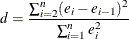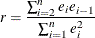The REG Procedure

Autocorrelation in Time Series Data

When regression is performed on time series data, the errors might not be independent. Often errors are autocorrelated; that is, each error is correlated with the error immediately before it. Autocorrelation is also a symptom of systematic lack of fit. The DW option provides the Durbin-Watson d statistic to test that the autocorrelation is zero:The value of d is close to 2 if the errors are uncorrelated. The distribution of d is reported by Durbin and Watson (1951). Tables of the distribution are found in most econometrics textbooks, such as Johnston (1972) and Pindyck and Rubinfeld (1981).

The sample autocorrelation estimate is displayed after the Durbin-Watson statistic. The sample is computed asThis autocorrelation of the residuals might not be a very good estimate of the autocorrelation of the true errors, especially if there are few observations and the independent variables have certain patterns. If there are missing observations in the regression, these measures are computed as though the missing observations did not exist.

Positive autocorrelation of the errors generally tends to make the estimate of the error variance too small, so confidence intervals are too narrow and true null hypotheses are rejected with a higher probability than the stated significance level. Negative autocorrelation of the errors generally tends to make the estimate of the error variance too large, so confidence intervals are too wide and the power of significance tests is reduced. With either positive or negative autocorrelation, least squares parameter estimates are usually not as efficient as generalized least squares parameter estimates. For more details, see Judge et al. (1985, Chapter 8) and the SAS/ETS User's Guide.

The following SAS statements request the DWPROB option for the U.S. population data (see Figure 97.53). If you use the DW option instead of the DWPROB option, then p-values are not produced.

proc reg data=USPopulation;
model Population=Year YearSq / dwProb;
run;


Figure 97.53: Regression Using DW Option

The REG Procedure
Model: MODEL1
Dependent Variable: Population

 Durbin-Watson D 1.191 0.005 0.995 22 0.323